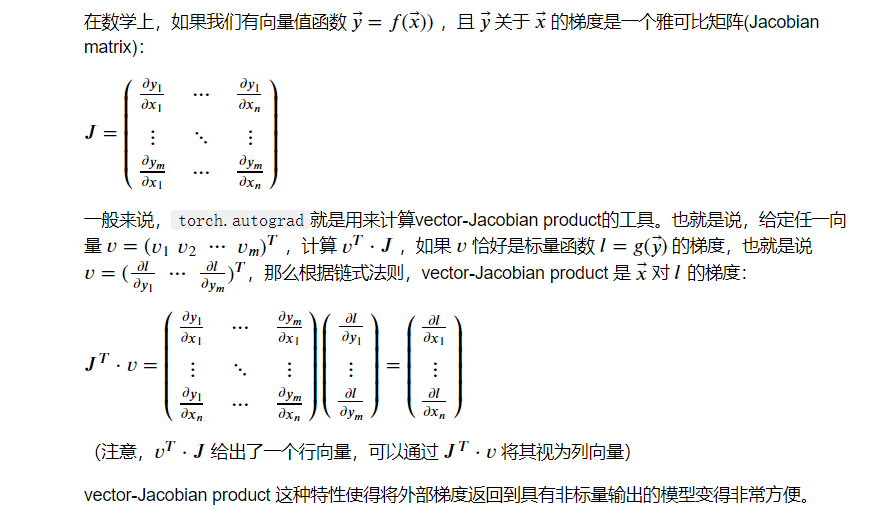PyTorch 中所有神经网络的核心是 `autograd` 包。

`autograd` 包为张量上的所有操作提供了自动求导。它是一个在运行时定义的框架，可以通过代码的运行来决定反向传播的过程，并且每次迭代可以是不同的。

## Tensor 张量

`torch.tensor`是这个包的核心类。

• 设置`.requires_grad``True`，会追踪所有对于该张量的操作。计算完成后调用`.backward()`，可以自动计算所有的梯度，并自动累计到`.grad`属性中

• 可以调用`.detach()`将该张量与计算历史记录分离，并禁止跟踪它将来的计算记录

• 为防止跟踪历史记录（和使用内存），可以将代码块包装在`with torch.no_grad(): `中。这在评估模型时特别有用，因为模型可能具有`requires_grad = True`的可训练参数，但是我们不需要梯度计算。

## Function类

`Tensor``Function` 互相连接并生成一个非循环图，它表示和存储了完整的计算历史。

leaf Tensors 叶张量

`Tensor`中有一属性`is_leaf`，当它为`True`有两种情况：

1. 按照惯例，`requires_grad = False` 的 Tensor
1. `requires_grad = True` 且由用户创建的 Tensor。这意味着它们不是操作的结果且`grad_fn = None`

``````x = torch.ones(2, 2, requires_grad=True)
print(x)
# Output:
# tensor([[1., 1.],
y = x + 2
print(y)
# Output:
# tensor([[3., 3.],
``````

``````>>> print(y.grad_fn)
``````

``````z = y * y * 3
out = z.mean()
print(z, out)
# Output:
# tensor([[27., 27.],
``````

`.requires_grad_( ... )` 可以改变现有张量的 `requires_grad`属性。 如果没有指定的话，默认输入的flag是 `False`

``````out.backward()
# Output:
# tensor([[4.5000, 4.5000],
#        [4.5000, 4.5000]])
````````````x = torch.randn(3, requires_grad=True)
y = x * 2
while y.data.norm() < 1000:
y = y * 2
print(y)
# Output:
# tensor([ 293.4463,   50.6356, 1031.2501], grad_fn=<MulBackward0>)
``````

``````v = torch.tensor([0.1, 1.0, 0.0001], dtype=torch.float)
y.backward(v)
# Output:
# tensor([5.1200e+01, 5.1200e+02, 5.1200e-02])
``````

``````print(x.requires_grad) # True

``````

### 推导

`grad_fn`中有一属性`next_functions`，例如

``````x = torch.rand(5, 5, requires_grad=True)
z = x**2 + y**3
# ((<PowBackward0 at 0x1426dc923c8>, 0), (<PowBackward0 at 0x1426dc92b48>, 0))
``````

``````xg = z.grad_fn.next_functions
x_leaf = xg.next_functions
``````

`AccumulateGrad`中有一个`.variable`指向叶子节点

``````x_leaf.variable
``````

### 流程

1. 当我们执行`z.backward()`时。这个操作将调用z里面的`grad_fn`属性，执行求导操作。
2. 这个操作将遍历`grad_fn``next_functions`，然后分别取出里面的`Function(AccumulateGrad)`，执行求导操作。这部分是一个递归的过程直到最后类型为叶子节点
3. 计算出结果以后，将结果保存到他们对应的`variable` 变量所引用的对象（x和y）的`grad`属性中
4. 求导结束。所有的叶节点的`grad`变量都得到了相应的更新

• `__init__` (optional)：如果这个操作需要额外的参数则需要定义这个Function的构造函数，不需要的话可以忽略。

• `forward()`：执行前向传播的计算代码

• `backward()`：反向传播时梯度计算的代码。 参数的个数和forward返回值的个数一样，每个参数代表传回到此操作的梯度。